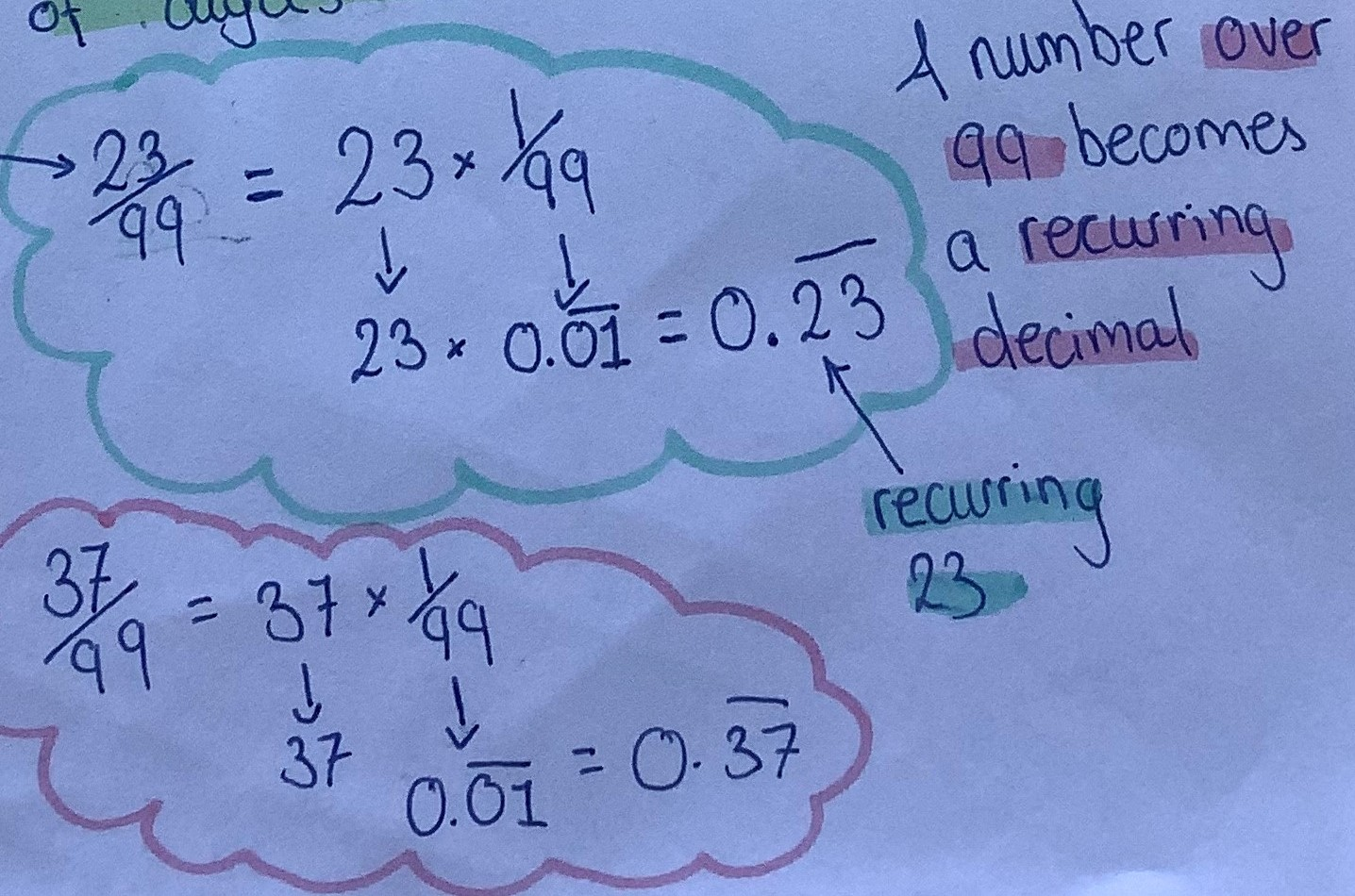#### You may also like### Repetitiously

Can you express every recurring decimal as a fraction?

# Tiny Nines

##### Age 14 to 16Challenge Level

Sanika from PSBBMS in India, ThÃ´ng-sahge from Tiffin School in the UK, Zofia from the Netherlands, Mahdi from Mahatma Gandhi International School in India and Turbo from Bangkok Patana School in Thailand all predicted the same decimal for $1\div9999$. This is some of Turbo's explanation:

Since $\frac19$ is $0.1111111111111”¦$ and $\frac{1}{99}$ is $0.01010101010101”¦$ and $\frac1{999}$ is $0.001001001001001”¦$ there is a pattern that follows these rules:

1. For every $1$, there is a certain amount of zeros following it (with the only exception of $\frac19.$
2. Depending on how many numerals in the denominator of the factor you wish to convert, the amount of numerals is one more than the number of zeroes you put before the one.

E.g.
$0.111111111111111”¦$ is $\frac19$ and since $\frac19$ has one [digit in its] denominator, you minus one away from the number one (the [number of digits in the] denominator) to get $0,$ therefore, there are no zeroes before the one.
$0.01010101010101”¦$ is $\frac1{99}$ and since $\frac1{99}$ has two [digits in its] denominator (the two $9$s), you minus one away from the $2$ (the [number of digits in the] denominator) to get one, therefore, there is $1$ zero before each one.
Using this method, we can get $\frac{1}{9999}$ equal $0.00010001000100010001”¦$ since there are four [digits in the denominator] from which we minus one to get the result of $3.$ So, there are $3$ $0$s before the one.

Turbo wanted to generalise algebraically. Turbo wrote:

$\frac1a = 0.b1b1b1b1b1b1”¦$
$a$ is how many denominator $9$ digits
$b$ is $a-1$ amount of $0$s.

Having read the rest of Turbo's working, we understand what Turbo means. Mahdi also wanted to generalise algebraically. What Mahdi wrote is easier to understand even without seeing the rest of Mahdi's working:
$$\frac1{\underbrace{99...00}_k} = 0.\underbrace{\dot{0}00...\dot{1}}_k$$

Sanika, Zofia and Mahdi found the decimals equivalent to the other fractions. This is some of Sanika's work:

Part A
\begin{align}\tfrac13 &= \tfrac19\times 3\\ &=0.1111...\times3\\ &= 0.3333...\end{align}

\begin{align}\tfrac1{33} &= \tfrac1{99}\times 3\\ &=0.010101...\times3\\ &= 0.030303...\end{align}

\begin{align}\tfrac1{333} &= \tfrac1{999}\times 3\\ &=0.001001001...\times3\\ &= 0.003003003...\end{align}

Part B
Similarly,
\begin{align}\tfrac1{11} &= \tfrac1{99}\times 9\\ &=0.010101...\times9\\ &= 0.090909...\end{align}

\begin{align}\tfrac1{111} &= \tfrac1{999}\times 9\\ &=0.001001001...\times9\\ &= 0.009009009...\end{align}

\begin{align}\tfrac1{1111} &= \tfrac1{9999}\times 9\\ &=0.00010001...\times9\\ &= 0.00090009...\end{align}

This is some of Zofia's work:Sanika explained how to extend this to any integer $n$:

$\tfrac n{99} = \tfrac1{99}\times n = 0.010101...\times n$

• if $n$ has one digit, replace all $1$s in $0.01010101...$ with $n$
• if $n$ has two digits then replace the zeros with the digit in the tens place and the $1$s with the digit in the units place
• if $n\gt99$ then do the following:
$\left[\left(n \text{ (mod }99)\right)\times 0.010101... \right] + \left[n - \left(n \text{ (mod } 99)\right)\div9\right]$
(Where $n \text{ (mod }99)$ means the remainder when $n$ is divided by $99$)

Mahdi gave an example which shows what Sanika means for the cases where $n\gt99$:

\begin{align}\tfrac{320}{99} &=3+\tfrac{23}{99}\\ &=3+0.\dot2\dot3\\ &=3.\dot2\dot3\end{align}

Mahdi also extended this to other similar fractions:

$\frac{n}{\underbrace{99..99}_k} = n\times0.\underbrace{\dot000...\dot1}_k$

SHORT CUT: Make $n$ [contain $k$ digits] by adding zeros in front of $n$

$\frac{n}{\underbrace{99..99}_k} = 0.\underbrace{\dot{n}}_k$

To prove that these recurring decimals are equivalent to the fractions that they are supposed to represent, look at the problem Repetitously.